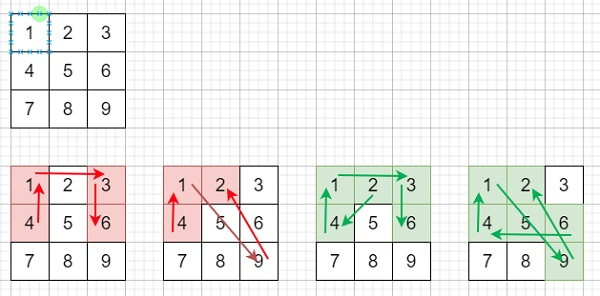# Android Unlock Patterns in C++

Suppose we have an Android 3x3 key lock screen and two integers m and n, the values of m and n are in range 1 ≤ m ≤ n ≤ 9, We have to count the total number of unlock patterns of the Android lock screen, which consist of minimum of m keys and maximum n keys.

The rule is like, each pattern must connect at least m keys and at most n keys. All the keys must be unique. If there is a line connecting two consecutive keys in the pattern passes through any other keys, the other keys must have previously selected in the pattern. Jumping through any key that is not selected that is not allowed. The order of keys used matters.So, if the input is like m = 1, n = 1, then the output will be 9

To solve this, we will follow these steps −

• Define an array skip of size: 10 x 10.

• Define a function dfs(), this will take node, len, an array visited,

• if len is same as 0, then −

• return 1

• visited[node] := true

• ret := 0

• for initialize i := 1, when i <= 9, update (increase i by 1), do −

• if visited[i] is false and (skip[node, i] is same as 0 or visited[skip[node, i]] is nonzero), then −

• ret := ret + dfs(i, len - 1, visited)

• visited[node] := false

• return ret

• From the main method do the following −

• fill skip with 0

• skip[1, 3] := skip[3, 1] := 2

• skip[1, 7] := skip[7, 1] := 4

• skip[3, 9] := skip[9, 3] := 6

• skip[7, 9] := skip[9, 7] := 8

• skip[4, 6] := skip[6, 4] := skip[2, 8] := skip[8, 2] := skip[3, 7] := skip[7, 3] := skip[1, 9] := skip[9, 1] := 5

• Define an array visited of size 10

• ret := 0

• for initialize i := m, when i <= n, update (increase i by 1), do −

• ret := ret + (dfs(1, i - 1, visited))

• ret := ret + (dfs(2, i - 1, visited))

• ret := ret + dfs(5, i - 1, visited)

• return ret

## Example

Let us see the following implementation to get better understanding −

Live Demo

#include <bits/stdc++.h>
using namespace std;
class Solution {
public:
int skip;
int dfs(int node, int len, vector<bool>& visited){
if (len == 0)
return 1;
visited[node] = true;
int ret = 0;
for (int i = 1; i <= 9; i++) {
if (!visited[i] && (skip[node][i] == 0 || visited[skip[node][i]])) {
ret += dfs(i, len - 1, visited);
}
}
visited[node] = false;
return ret;
}
int numberOfPatterns(int m, int n){
memset(skip, 0, sizeof(skip));
skip = skip = 2;
skip = skip = 4;
skip = skip = 6;
skip = skip = 8;
skip = skip = skip = skip = skip = skip = skip = skip = 5;
vector<bool> visited(10);
int ret = 0;
for (int i = m; i <= n; i++) {
ret += (dfs(1, i - 1, visited) * 4);
ret += (dfs(2, i - 1, visited) * 4);
ret += dfs(5, i - 1, visited);
}
return ret;
}
};
main(){
Solution ob;
cout << (ob.numberOfPatterns(1,1));
}

## Input

1, 1

## Output

9